#### A charge Q  is uniformly distributed over the surface of non conducting disc of radius  R. The disc rotates  about an axis perpendicular to its plane and passing through its centre with an angular velocity  . As a result of this rotation a magnetic field of induction B is obtained at the centre of the disc. If we keep both the amount of charge placed on the disc and its angular velocity to be constant and vary the radius of the disc then the variation of the magnetic induction at the centre of the disc will be represented by the figure .Option: 1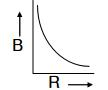Option: 2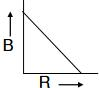Option: 3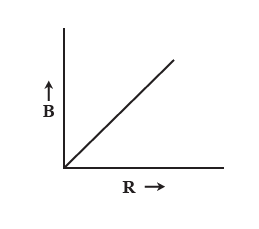Option: 4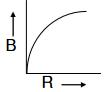Consider an elementary ring of radius  , and thickness of a disc as shown in the figure.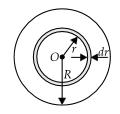Charge on the ring,

Current due to the rotation of charge on the ring is

The magnetic field at the centre due to the ring element

The magnetic field at the centre due to the whole disc

Since, and are constants

Hence variation of B with R should be a rectangular hyperbola as represented in